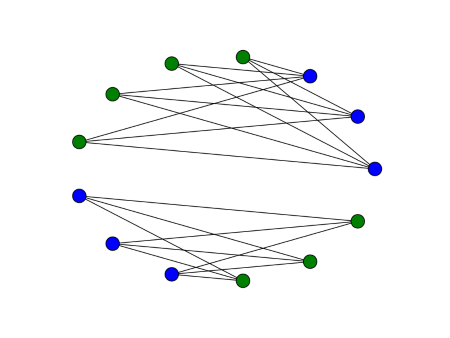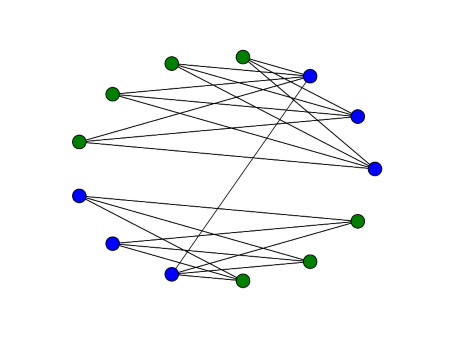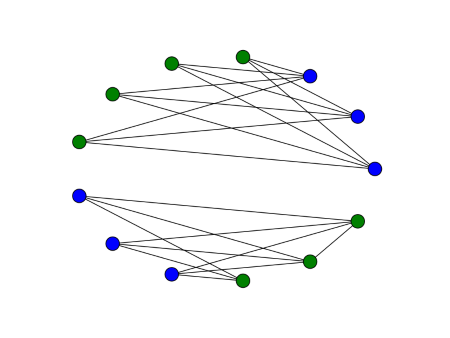# Bipartite graphs and the signless Laplacian

The vertices of a bipartite graph can be divided into two sets where edges only go from one set to the other; there are no edges within each set. For example, in the graph below edges connect blue to green nodes, but not blue to blue or green to green.In this example there are two connected bipartite components. There is a variation on the graph Laplacian called the signless Laplacian whose eigenvalues can tell you how many bipartite components a graph has.

The graph Laplacian is LD – A where D is the diagonal matrix whose entries are the degrees of each node and A is the adjacency matrix. The signless Laplacian is QDA. Since D and A have only non-negative entries, so does Q.

The smallest eigenvalue of the Laplacian L is always zero, and the second smallest eigenvalue is positive if and only if the graph is connected. The smallest eigenvalue of the signless Laplacian Q is positive if and only if the graph is connected and bipartite. The multiplicity of zero as an eigenvalue equals the number of connected bipartite components.

The graph above has two connected bipartite components. Zero is a double eigenvalue of the signless Laplacian, and the next eigenvalue is 0.2603.

Next we connect two of the blue nodes. Now the graph is connected and bipartite.Zero is now a single eigenvalue, and the second eigenvalue is 0.0968. This second eigenvalue is positive because there is only one component now, but it is small because the graph can almost be separated into two bipartite components.

Next we remove the edge connecting the two components, but we draw an edge between two of the green vertices.Now there are two components, but only one of them is bipartite. The smallest eigenvalue is zero, with multiplicity 1, and the second eigenvalue is 0.2015.

Next we repeat the examples above with more edges. Now each component of the graph is a complete bipartite graph.The smallest eigenvalue is 0, with multiplicity 2. But now the next eigenvalue is 3, This value is larger than before because the components are better connected.

As before, we now join two of the blue nodes.Now there is one bipartite component. The smallest eigenvalue is 0 with multiplicity 1. The second eigenvalue is 0.2103.

Finally, we remove the edge connecting the two components and connect two of the green vertices.Now the smallest eigenvalue is 0 with multiplicity 1. While there are two components, there’s only one bipartite component. The next eigenvalue is 0.4812.

## One thought on “Bipartite graphs and the signless Laplacian”

1. In “The smallest eigenvalue of the signless Laplacian Q is positive if and only if the graph is connected and bipartite” the word “positive” should be “zero” and “connected” is not needed. Unless you wanted to say something else.

Aside from that, I’m curious why do you care about the number of bipartite connected components from an applied point of view? Any examples where this comes up in applications?